# Polygon

A polygon is a closed plane figure formed by three or more line segments. The following are a few examples.

The line segments that form a polygon are called sides. Two connected sides form an angle at a point called a vertex. A diagonal is a line segment joining two non-consecutive vertices. In the polygon below, AB, BC, CD, and AD are four sides. They form four angles: ∠A, ∠B, ∠C, and ∠D. AC and BD are two diagonals.

There are many ways to classify polygons; the following are some of them.

## Regular and irregular polygons

### Regular polygon

A regular polygon is a polygon in which all sides have equal length (equilateral) and all angles have equal measure (equiangular). Below are some examples.

### Irregular polygon

An irregular polygon has sides or angles that are not congruent, as shown below.

## Convex and concave polygons

Polygons can be classified as either convex or concave.

### Convex polygon

If all the interior angles of a polygon are less than 180°, it is convex. A regular polygon is always convex. The following are a few examples.

### Concave polygon

If one or more interior angles of a polygon are larger than 180°, it is concave. A concave polygon is always an irregular polygon. The following are a few examples. The interior angles larger than 180° are marked with a red arc.

## Classifying polygons by their number of sides

Polygons are commonly classified based on the number of sides they have. In general, a polygon with n-number of sides is called an n-gon. Some important polygons have specific names, such as triangles, pentagons, hexagons, etc. The following are some examples.

Polygon# of sidesShape
Triangle3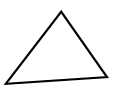Quadrilateral4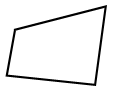Pentagon5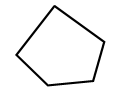Hexagon6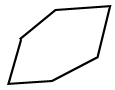Octagon8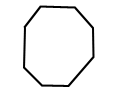There are many other polygons, and each polygon above can be further classified. For example, a triangle can be further classified as an acute, obtuse, or right triangle. Learn more about these polygons by navigating this website.